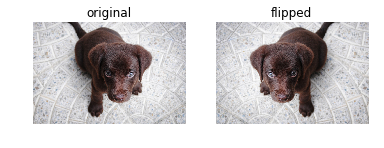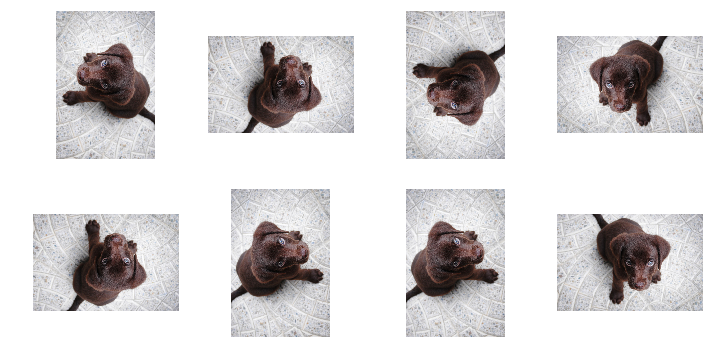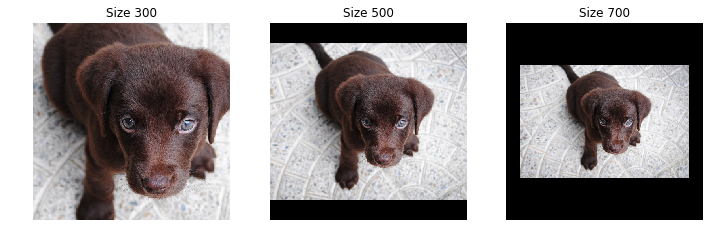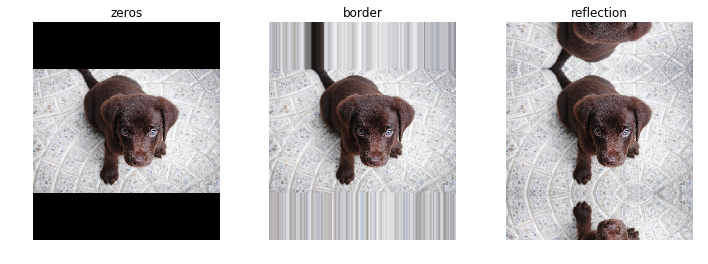Transforms to apply data augmentation in Computer Vision
from nbdev.showdoc import *

img = PILImage(PILImage.create(TEST_IMAGE).resize((600,400)))


## General architecture

### classRandTransform[source]

RandTransform(p=1.0, nm=None, before_call=None, **kwargs) :: Transform

A transform that before_call its state at each __call__

As for all Transform you can pass encodes and decodes at init or subclass and implement them. You can do the same for the before_call method that is called at each __call__. Note that to have a consistent state for inputs and targets, a RandTransform must be applied at the tuple level.

By default the before_call behavior is to execute the transform with probability p (if subclassing and wanting to tweak that behavior, the attribute self.do, if it exists, is looked for to decide if the transform is executed or not).

#### RandTransform.before_call[source]

RandTransform.before_call(b, split_idx)

before_call the state for input b

def _add1(x): return x+1
start,d1,d2 = 2,False,False
for _ in range(40):
t = dumb_tfm(start, split_idx=0)
if dumb_tfm.do: test_eq(t, start+1); d1=True
else:           test_eq(t, start)  ; d2=True
assert d1 and d2


## Item transforms

#### Image.flip_lr[source]

Image.flip_lr(x:Image)

#### TensorImageBase.flip_lr[source]

TensorImageBase.flip_lr(x:TensorImageBase)

#### TensorPoint.flip_lr[source]

TensorPoint.flip_lr(x:TensorPoint)

#### TensorBBox.flip_lr[source]

TensorBBox.flip_lr(x:TensorBBox)

_,axs = subplots(1,2)
show_image(img, ctx=axs, title='original')
show_image(img.flip_lr(), ctx=axs, title='flipped');### classFlipItem[source]

FlipItem(p=0.5) :: RandTransform

Randomly flip with probability p

tflip = FlipItem(p=1.)
test_eq(tflip(bbox,split_idx=0), tensor([[1.,0., 0.,1]]) -1)


#### PILImage.dihedral[source]

PILImage.dihedral(x:PILImage, k)

#### TensorImage.dihedral[source]

TensorImage.dihedral(x:TensorImage, k)

#### TensorPoint.dihedral[source]

TensorPoint.dihedral(x:TensorPoint, k)

#### TensorBBox.dihedral[source]

TensorBBox.dihedral(x:TensorBBox, k)

By default each of the 8 dihedral transformations (including noop) have the same probability of being picked when the transform is applied. You can customize this behavior by passing your own draw function. To force a specific flip, you can also pass an integer between 0 and 7.

### classDihedralItem[source]

DihedralItem(p=0.5) :: RandTransform

Randomly flip with probability p

_,axs = subplots(2, 4)
for ax in axs.flatten():
show_image(DihedralItem(p=1.)(img, split_idx=0), ctx=ax)## Resize with crop, pad or squish

### classPadMode[source]

PadMode(*args, **kwargs)

All possible padding mode as attributes to get tab-completion and typo-proofing

#### TensorBBox.crop_pad[source]

TensorBBox.crop_pad(x:Image'>), sz, tl=None, orig_sz=None, pad_mode='zeros', resize_mode=2, resize_to=None)

#### TensorPoint.crop_pad[source]

TensorPoint.crop_pad(x:Image'>), sz, tl=None, orig_sz=None, pad_mode='zeros', resize_mode=2, resize_to=None)

#### Image.crop_pad[source]

Image.crop_pad(x:Image'>), sz, tl=None, orig_sz=None, pad_mode='zeros', resize_mode=2, resize_to=None)

### classCropPad[source]

CropPad(size, pad_mode='zeros', **kwargs) :: Transform

Center crop or pad an image to size

_,axs = plt.subplots(1,3,figsize=(12,4))
for ax,sz in zip(axs.flatten(), [300, 500, 700]):_,axs = plt.subplots(1,3,figsize=(12,4))### classRandomCrop[source]

RandomCrop(size, **kwargs) :: RandTransform

Randomly crop an image to size

### classOldRandomCrop[source]

OldRandomCrop(size, pad_mode='zeros', enc=None, dec=None, split_idx=None, order=None) :: CropPad

Randomly crop an image to size

_,axs = plt.subplots(1,3,figsize=(12,4))
f = RandomCrop(200)
for ax in axs: show_image(f(img), ctx=ax);